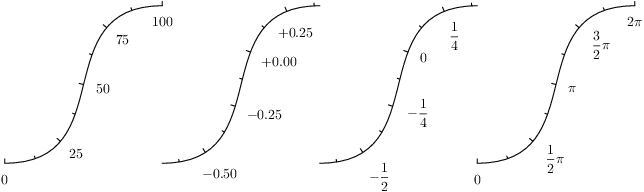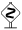# PyX — Example: axis/texter.py

0.9 KB
64.8 KB
51.2 KB
25.7 KB
44.1 KB

## Texter: Controlling the formatting of tick labelsimport math
from pyx import *
from pyx.graph import axis

texter1 = axis.texter.decimal(plus="+", minus="-", equalprecision=1)
texter2 = axis.texter.rational()

class piaxis(axis.linear):

def __init__(self, divisor=math.pi,
texter=axis.texter.rational(suffix=r"\pi"), **kwargs):
axis.linear.__init__(self, divisor=divisor, texter=texter, **kwargs)

p1 = path.path(path.moveto(0, 0), path.curveto(3, 0, 1, 4, 4, 4))
p2 = p1.transformed(trafo.translate(4, 0))
p3 = p2.transformed(trafo.translate(4, 0))
p4 = p3.transformed(trafo.translate(4, 0))

c = canvas.canvas()
c.insert(axis.pathaxis(p1, axis.linear(min=0, max=1e2)))
c.insert(axis.pathaxis(p2, axis.linear(min=-0.7, max=0.4, texter=texter1)))
c.insert(axis.pathaxis(p3, axis.linear(min=-0.7, max=0.4, texter=texter2)))
c.insert(axis.pathaxis(p4, piaxis(min=0, max=2*math.pi)))
c.writeEPSfile("texter")
c.writePDFfile("texter")
c.writeSVGfile("texter")


### Description

Texters do the formatting of the tick labels: They create the strings which are then passed to the painter. You can choose between fractions and decimal numbers with/without an exponential part. The texter should not be mixed up with the painter: The painter is afterwards doing the TeXing and finally creates the labels -- the texter prepares the string that is to be processed by TeX.

The first example shows the default behavior, which chooses either decimal and exponential formatting.

The second example is for special purpose only (because it is ugly), in case that it is strictly necessary to have the same width of all labels.

The third example shows how to create fractions instead of decimal numbers.At the rightmost axis we also show how to create a special piaxis. Here, some axis parameters are altered to fit an pi-scaled axis nicely. Futhermore, instead of just creating a special axis instance by setting the divisor and texter parameters, a piaxis class is created which just alters the defaults of the linear axis it is based at.